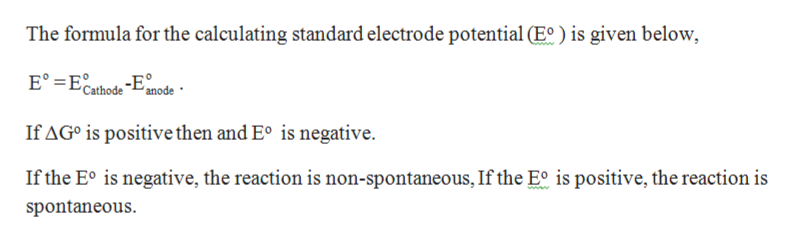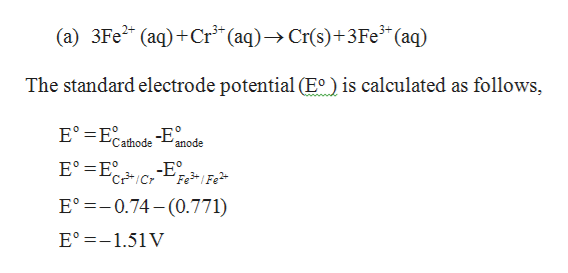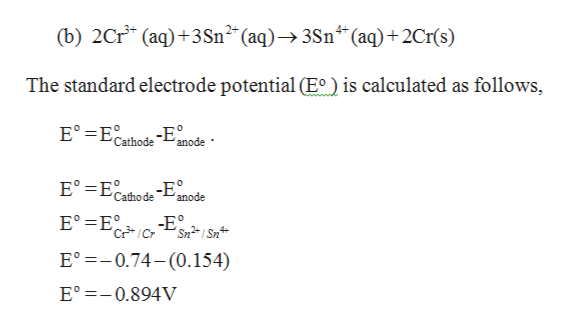# Which of the following reactions will occur spontaneously as written? A. 3Fe2+(aq) + Cr3+(aq) -> Cr (s)  3 Fe3+ (aq)B. 2Cr3+ (aq) + 3Sn2+ (aq) -> 3Sn4+ (aq) + 2Cr (s)C. Sn4+ (aq) + Fe2+ (s) -> Sn2+ (aq) + Fe (s)D. Sn2+ (aq) + Fe2+ (s) -> Sn4+ (aq) + Fe 3+ (aq)E. 2Cr (s) + 3Fe2+ (s) -> 3Fe (s) + 2Cr3+ (aq)

Question
950 views

Which of the following reactions will occur spontaneously as written?

A. 3Fe2+(aq) + Cr3+(aq) -> Cr (s)  3 Fe3+ (aq)

B. 2Cr3+ (aq) + 3Sn2+ (aq) -> 3Sn4+ (aq) + 2Cr (s)

C. Sn4+ (aq) + Fe2+ (s) -> Sn2+ (aq) + Fe (s)

D. Sn2+ (aq) + Fe2+ (s) -> Sn4+ (aq) + Fe 3+ (aq)

E. 2Cr (s) + 3Fe2+ (s) -> 3Fe (s) + 2Cr3+ (aq)

check_circle

Step 1

The relationship between ΔGo and Eo is shown below,

ΔGo = -nFEohelp_outlineImage TranscriptioncloseThe formula for the calculating standard electrode potential (E° is given below 'anode If AGo is positive then and E° is negative If the Eo is negative, the reaction is non-spontaneous, If the Eo is positive, the reaction is spontaneous fullscreen
Step 2

Eo of the given reaction is negative, therefore the reaction is non-spontaneous.help_outlineImage TranscriptioncloseЗFe* (аq) +Сr* (aq) > Cr(s)+3Fe*" (aq) (а) The standard electrode potential (Eo is calculated as follows, E° Ehode -E Cat anode E° E E C 'Fe3Fe Cr E -0.74-(0.771) Eo 1.51 V fullscreen
Step 3

Eo of the given reaction is negative, therefore...help_outlineImage Transcriptionclose(b) 2Cr (aq)3Sn2 (aq)->3Sn*(aq) +2Cr(s) The standard electrode potential (E° is calculated as follows, E° Ecathode-Eode Cathode anode E° =ErSnSn -E C E° 0.74-(0.154) E° 0.894V fullscreen

### Want to see the full answer?

See Solution

#### Want to see this answer and more?

Solutions are written by subject experts who are available 24/7. Questions are typically answered within 1 hour.*

See Solution
*Response times may vary by subject and question.
Tagged in

### General Chemistry